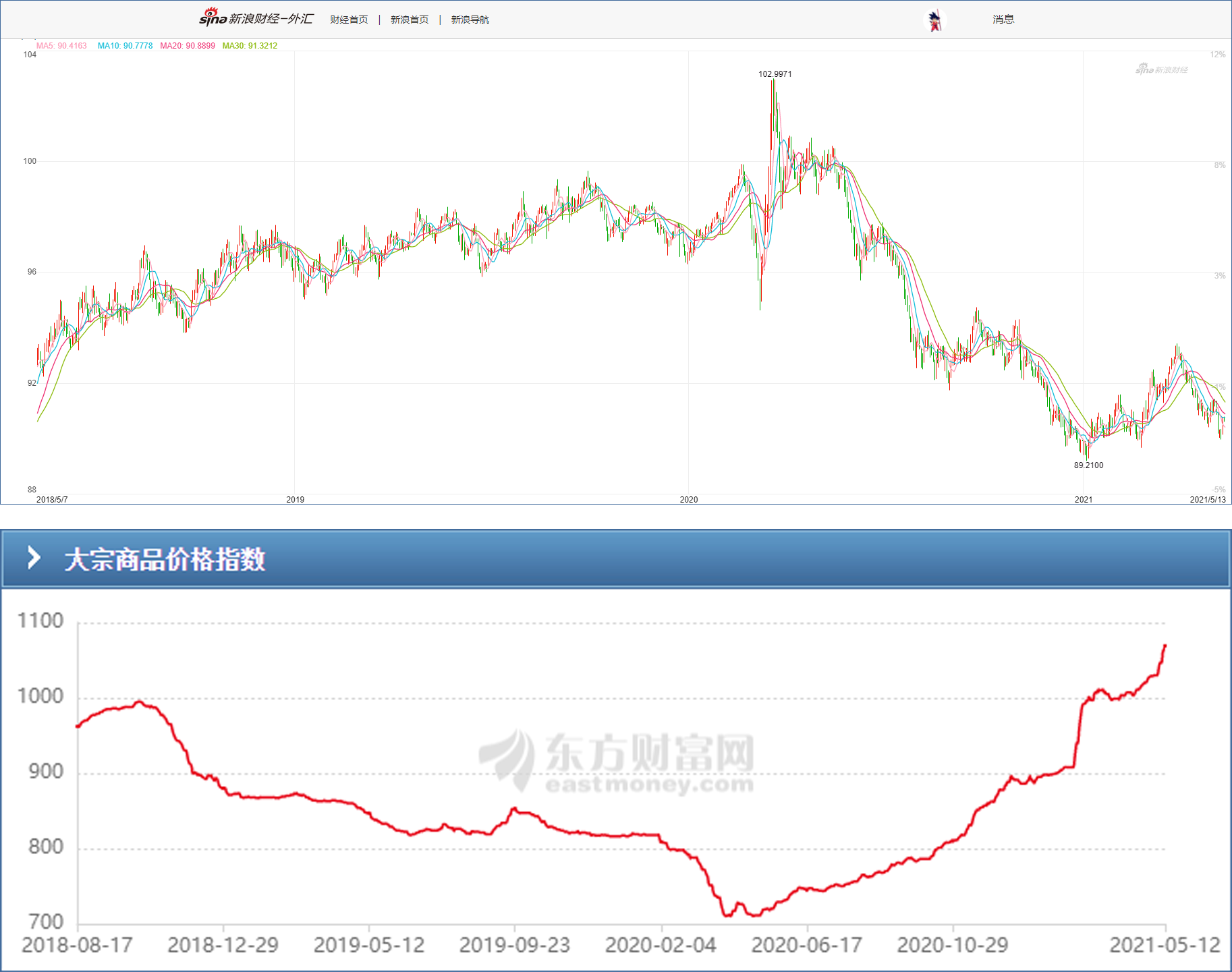Loading ...

# CTA策略之商品期货黑色系多头择时策略

Author: Hukybo, Date: 2021-05-08 16:44:58
Tags:

#### 二、大宗商品上涨原因

2、货币供应量 2020年疫情之后，全球主要经济体央行集体放水，尤其是美欧日三国M2同比增速超过20%，在各国央行宽松政策不变的情况下，市场通胀预期仍然强烈。

3、全球经济复苏 随着各国疫苗接种顺利，经济回暖，大宗商品需求反弹，全球制造业采购经理指数（PMI）持续扩张，制造业的复苏对工业品价格形成支撑。

#### 三、美元指数与大宗商品

2021年与我们日常生活息息相关的上游物资，不知不觉均出现明显上涨。正如巴菲特所言这一切都拜美联储宽松的货币和财政政策所赐，由于疫情期间美国出台的一系列天量刺激措施，全球的经济复苏已经裹挟着通胀奔涌而来。图片来源：新浪财经、东方财富

MV=PQ

• M：货币总量
• V：货币周转速率
• P：商品价格
• Q：商品数量

#### 五、策略逻辑及实现

• 计算前4根K线最高价与开盘价的差的均值
• 计算HO1、HO2、HO3、HO4均值
• 计算多头开仓信号
• 计算多头平仓信号
• 下单交易

``````HO1 := REF(H, 1) - REF(O, 1);
HO2 := REF(H, 2) - REF(O, 2);
HO3 := REF(H, 3) - REF(O, 3);
HO4 := REF(H, 4) - REF(O, 4);
HTO := (HO1 + HO2 + HO3 + HO4) / 4;
``````

``````LINE := O - HTO * N;
``````

``````D1 := REF(C, 1) < REF(C, 2);
D2 := REF(C, 2) < REF(C, 3);
D3 := REF(C, 3) < REF(C, 4);
D := D1 && D2 && D3 && C > LINE;
``````

``````K1 := REF(C, 1) > REF(C, 2);
K2 := REF(C, 2) > REF(C, 3);
K3 := REF(C, 3) > REF(C, 4);
K := K1 && K2 && K3 && C <= LINE;
T1 := BARSLAST(BARSLAST(K) < BARSLAST(D));
DT := IFELSE(T1 <= REF(T1, 1), 0, (C - VALUEWHEN(T1 = 1, C)));
``````

``````D, BK;
T1 < 5 && T1 > 3 && DT < 7, SP;
``````

#### 六、策略回测

• 回测开始日期：2015-02-22
• 回测结束日期：2021-05-12
• 数据品种：螺纹钢指数
• 数据周期：日线
• 滑点：开平仓各2跳

```(*backtest
start: 2015-02-22 00:00:00
end: 2021-05-07 00:00:00
period: 1d
basePeriod: 1d
exchanges: [{"eid":"Futures_CTP","currency":"FUTURES"}]
args: [["ContractType","rb000",126961]]
*)

HO1 := REF(H, 1) - REF(O, 1);
HO2 := REF(H, 2) - REF(O, 2);
HO3 := REF(H, 3) - REF(O, 3);
HO4 := REF(H, 4) - REF(O, 4);
HTO := (HO1 + HO2 + HO3 + HO4) / 4;
LINE := O - HTO * N;
D1 := REF(C, 1) < REF(C, 2);
D2 := REF(C, 2) < REF(C, 3);
D3 := REF(C, 3) < REF(C, 4);
D := D1 && D2 && D3 && C > LINE;
K1 := REF(C, 1) > REF(C, 2);
K2 := REF(C, 2) > REF(C, 3);
K3 := REF(C, 3) > REF(C, 4);
K := K1 && K2 && K3 && C <= LINE;
T1 := BARSLAST(BARSLAST(K) < BARSLAST(D));
DT := IFELSE(T1 <= REF(T1, 1), 0, (C - VALUEWHEN(T1 = 1, C)));
D, BK;
T1 < 5 && T1 > 3 && DT < 7, SP;
```

More